# Reaction rate and order

| Home | | Pharmaceutical Drugs and Dosage | | Pharmaceutical Industrial Management |

## Chapter: Pharmaceutical Drugs and Dosage: Chemical kinetics and stability

The rate of a reaction is the amount or concentration of a degradation product formed or the reactant lost per unit time. The rate of a reaction is described by a rate equation.

Reaction rate and order

The rate of a reaction is the amount or concentration of a degradation product formed or the reactant lost per unit time. The rate of a reaction is described by a rate equation. For example, for a hypothetical reaction,

aA + b B mM + nN                        (7.1)

where:

A and B are the reactants

M and N are the products

a, b, m, and n are the stoichiometric coefficients (number of moles partici-pating in the reaction) for the corresponding reactant or product

The rate of this reaction can be described in terms of rate of disappearance of A or B or the rate of appearance of M or N, which are all interrelated. Thus,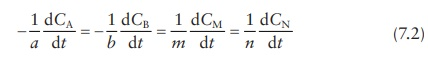Where, dCA is the change in the concentration of reactant A over a period of time dt, and respectively, for all reactants and products in the equation.

The rate equation, describing the rate of formation of a product or the rate of disappearance of a reactant, for this reaction can be written as: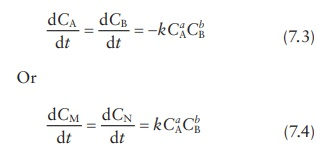where k is the rate constant.

The negative sign associated with the reactants indicates their rate of dis-appearance from the system; that is, the concentration after time period, t, is lower than the starting concentration. The rate equation for the products does not carry the negative sign, since it indicates the rate of formation or appearance of products in the system; that is, the concentration after time period, t, is higher than the starting concentration. The rate constant, k, is positive in both rate equations.

The order of a reaction is the sum of powers to which the concentra-tion terms of the reactants are raised in the rate equation. The order of a reaction can also be defined with respect to a given reactant. Hence, in the aforementioned example, the order of the reaction with respect to reactant A is a, order with respect to reactant B is b, and the overall order of the reaction is a + b.

Identifying the order of a reaction helps understand the dependence of the reaction on the concentrations of starting materials. Thus, reactions can be of zero order (indicating independence to reactant concentrations), first order (indicating that reaction rate is proportional to the first power of one of the reactants), second order (indicating that reaction rate is propor-tional to the first power of two of the reactants or the second power of one of the reactants), or higher order.

For example, for the base-catalyzed hydrolysis of an ester in the reaction,

CH 3COOC2H 5 + NaOH CH3COONa + C2 H5OH

Reaction rate is defined as:The reaction rate equation is:

Rate = k[ CH3COOC2H5 ][ NaOH]                    (7.6)

The order of this equation is 1 + 1 = 2, since both reactants are raised to the power of one in the rate equation.

The number of molecules taking part in a reaction is called the molecularity of a reaction. The molecularity of a reaction is determined by the mechanism of a reaction and is expressed in the reaction equation. The order of a reaction may or may not be same as the molecularity of a reaction. In the aforemen-tioned example, the molecularity of the reaction is 2, which is same as the order of the reaction.

## Pseudo-nth order reactions

The observed order of a reaction may sometimes be different than the sum of stoichiometric coefficients of reactants. The rate of a reaction may sometimes be independent of the concentration of one of the reactants, even though this reactant is consumed during the reaction. For example, if one of the two reactants is the solvent in which the other reactant is dis-solved at low concentration, such as an aqueous solution of a hydrolytically sensitive drug, the order of the reaction may be independent of the solvent concentration—the reactant present in a significantly higher concentration. Such reactions are termed as pseudo-nth order reactions. Thus, a truly sec-ond-order reaction, such as equimolar reaction of an ester compound with water in an aqueous solution, that presents itself as a first-order reaction is termed a pseudo-1st order reaction.

For example, for the hydrolysis of a dilute solution of ethyl acetate,

CH3COOCH2CH3 + H2O CH3COOH + CH3CH2OH

Reaction rate is defined as:The reaction rate equation is:

Rate = k[ CH3COOC2H5 ]                  (7.8)

The order of this equation is 1, since only one of the reactants is involved in the rate equation. Nevertheless, the molecularity of this reaction is 2, since two molecules are involved in the mechanism of the reaction and are expressed in the reaction equation. Pseudo-order reactions are typically the cases where the molecularity of a reaction is not the same as the order of a reaction.

## Determining the order of a reaction

The order of a reaction is determined experimentally, while molecularity of a reaction–which determines the equation–is determined by a thorough understanding of the reaction mechanism. The order of a reaction can be experimentally determined by one of the several methods:

1. Initial rate method. Initial rate of a reaction is measured for a series of reactions with varying concentrations of reactants to determine the power to which the reaction rate depends on the concentration of each reactant. Only the initial rate is measured to ensure that the reac-tant’s concentration is the predominant influence on the reaction rate.

As a reaction proceeds, the reaction rate can be influenced by changes in reaction conditions, such as accumulation of product or by-product of a reaction. Thus, the measurement of only the initial rate of a reac-tion provides a robust way to quantitate the dependence of reaction rate on the concentration of reactant(s).

2. Integrated rate law method. The concentration–time data of a reaction can be used to assess how the rate of a reaction changes as a function of reactant concentration. This plot is compared to theoretical predic-tions made by integrated rate equations, discussed later in this chapter, to infer reaction order.

3. Graph method. Similar to the integrated rate law method, this method plots the concentration–time profile of a reaction graphically to check fit to different reaction order kinetics.

4. Half-life method. The dependence of half-life, the time it takes for the reactant concentration to reach half of the measured initial concentration, on the initial concentration of the reactant is different for reactions of different orders. Half-lives of reactants can be determined experimentally and compared to theoretical predictions to determine reaction order.

## Zero-order reactions

A zero-order reaction is one in which the reaction rate is independent of the concentration(s) of the reactant(s). The rate of change of concentration of reactant(s) or product(s) in a zero-order reaction is constant and indepen-dent of the reactant concentration. Many decomposition reactions in the solid phase or in suspensions follow zero-order kinetics.

The reaction rate for most zero-order reactions depends on some other factor, such as absorption of light for photochemical reactions or the inter-facial surface area for heterogeneous reactions (i.e., reactions that happen at the solid–liquid, liquid–gas, or solid–gas interface). Thus, the slowest or the rate-determining factor of the reaction is different than the concentration(s) of reactant(s) for zero-order reactions.

Rate equation

Reaction rate of a zero-order reaction is constant and independent of the reactant concentration. Thus, the rate expression for the change in reactant concentration, C, with time, t, for a zero-order reaction (Figure 7.1) can be written as:Figure 7.1 Zero-order kinetics. Plot of concentration, C, versus time, t.

where k0 is the rate constant for a zero-order reaction. Thus, the change in the concentration of the reactant depends only on the time multiplied by a constant value, k0.

Integrating this equation from concentration C0 at time = 0 to concentra-tion = Ct at time = t,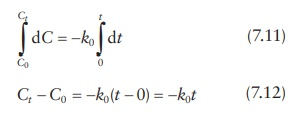Ct C0 = −k0 (t 0) = −k0t                     (7.12)

Thus, the rate equation (Figure 7.1) is:

Ct = C0 k0t                                           (7.13)

This is a linear equation of the form, y = mx + c. Thus, a plot of concentra-tion, Ct, on the y-axis against time, t, on the x-axis (Figure 5.1) is linear, with a slope of –k0 (downward slope of the line is indicated by the negative sign) and y-axis intercept of C0.

Half-life

Scientists are frequently interested in the time required for the reduction of a given proportion of starting drug concentration. For example, a drug’s shelf life is defined in terms of the time taken for the reduction of labeled drug concentration to its 90% level. The half-life (t1/2) of a reaction is defined as the time required for one-half of the material to decompose. Thus, con-centration of a reactant at its half-life (Ct1/2) is defined as half of the initial concentration (C0); that is:

Ct1/2 = C0 / 2                       (7.14)

Thus, for a zero-order reaction,

Ct 1/2 = C0 k0t1 /2                  (7.15)

C0/ 2 = C0k0t1/2               (7.16)

After rearranging, the half-life expression (Figure 7.1) for a zero-order reaction is:## First-order reactions

A first-order reaction is one in which the rate of reaction is directly proportional to the concentration of one of the reactants. Many decomposition reactions in the solid phase or in suspensions follow first-order kinetics. In a first-order reaction, concentration decreases exponentially with time, with the reaction rate slowing down progressively as the reactant is consumed in the reaction.

Rate equation

The rate of disappearance of the reactant is the concentration of the reactant multiplied by a constant. Thus, the first-order rate equation (Figure 7.2) is:Figure 7.2 First-order kinetics. Plot of concentration, C, against time, t (A), and plot of natural logarithm of the concentration, C, against time, t.Where, C is the reactant concentration at time t, and k is the first-order rate constant.

This equation can be rearranged as:Integrating this equation from concentration C0 at time = 0 to concentration = Ct at time = t,Solving this integral,

ln C lnC0 = −k(t 0) = −kt                 (7.21)

This equation can also be expressed as:Thus, the rate equation (Figure 7.2) is:

ln C = lnC0 kt                                   (7.22)

Or, in the exponential form, the rate equation can be expressed as:

C = C0ekt                                                                     (7.23)

Alternatively, by converting ln to the log base 10 (log10),

C = C010 kt/2.303                                (7.24)

Thus, in a first-order reaction, the concentration decreases exponentially with time (Figure 7.2). A plot of log or ln of concentration against time is a straight line, whose slope provides the rate constant, k.

Half-life

Half-life, t1/2, is defined as the time for the drug concentration to get to half of its original concentration (C0); that is, Ct = C0/2. This can be derived from the rate equation as: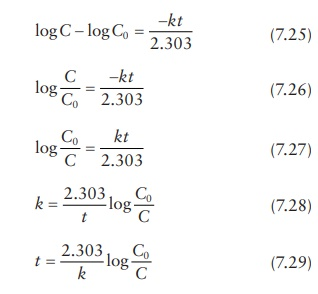Thus, the half-life of the reactant in a first-order reaction is given by: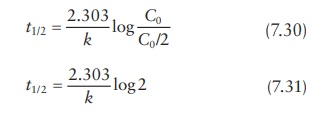Hence, the half-life expression (Figure 7.2) is:

t1/2 = 0.693/ k                         (7.32)

For a reaction observing first-order kinetics, the half-life, t1/2, or the time to any proportional reduction in concentration (e.g., t0.9, i.e., time to 90% of initial concentration), is a constant number and independent of the initial reactant concentration, C0.

## Second-order reactions

Bimolecular reactions, reactions involving two different molecules A and B, involve reactions of two molecules.

A + B Products

The rates of bimolecular reactions are frequently described by a second-order equation. The rate of change in the concentrations of products and reactants in second-order reactions is proportional either to the second power of the concentration of a single reactant or to the first powers of the concentrations of two reactants.

When the speed of the reaction depends on the concentrations of A and B, with each term raised to the first power, the rate of decomposition of A is equal to the rate of decomposition of B and both are proportional to the product of the concentrations of the reactants. This can be expressed as the rate equation:Rate equation

Assuming that the initial concentrations of A and B are same, that is, C0, and their concentration after time, t, is C, the rate equation can be written as: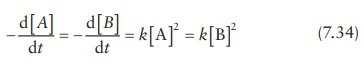Or, using their concentration value, C, the rate expression (Figure 7.3) is:Figure 7.3 Second-order kinetics. Plot of the reciprocal of the concentration, C, against time, t.

Integrating,Or, the rate equation (Figure 7.3) is: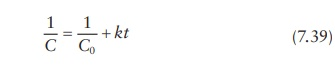Half-life

In a second-order reaction, the time to reach a certain fraction of the initial concentration (such as t1/2 or t0.90) is dependent on the initial concentration. The half-life is defined as: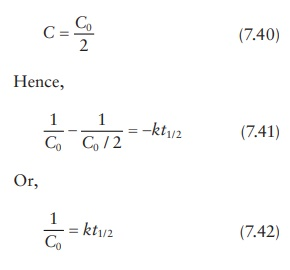Thus, the half-life expression (Figure 7.3) is:Hence, for a second-order reaction, t1/2 decreases with increasing initial concentration of the two reactants. This is consistent with the molecular mechanism of intermolecular reactions, where the two molecules must col-lide and react with each other for the reaction to happen. Thus, higher initial concentration increases the probability of collision and reaction between the molecules of two different types, increasing the probability and rate of the reaction.

## Complex reactions

Often, a drug undergoes more than one chemical reaction or a series of reactions in the same environment. Such complex reactions can be exemplified by reversible, parallel, or consecutive reactions. In such cases, the experimental methods for detection of reaction rates usually have limi-tations in that each reaction intermediate and product may not be detected or accurately quantitated. Thus, one may be quantitating a product whose concentration is impacted by the starting material or drug concentration in a complex manner. Understanding how such complexities might be linked to the kinetics of reactions can help delineate the mechanisms of degradation of drugs. This section describes the predicted kinetics of com-plex reactions.

### Reversible reactions

Reversible reactions are bidirectional; that is, the product can convert back to the reactant. The rate constant of the forward reaction can be designated by k1, and the rate constant of the reverse reaction can be des-ignated by k−1.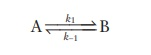Assuming first-order reaction in either direction, the rate of the forward reaction at equilibrium is described by:The rate of the forward reaction may not equal the rate of the reverse reaction. In fact, the rate of the forward and the reverse reactions may be affected by different environmental conditions. Equilibrium reactions are characterized by a constant ratio of the concentration of reactants and products, without regard to their absolute concentration.

### Parallel reactions

Parallel reactions involve two or more simultaneous reaction pathways for a reactant. For example,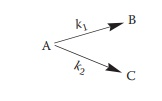Assuming first-order kinetics for both reactions, the individual reaction rates and the rates of formation of individual products are defined by the individual rate equations:The overall rate of degradation of a reactant is given by:

Rate = − d[A] / dt = k1[A] + k2[A] = (k1 + k2)[A] = kobs[A]                   (7.47)

where kobs is the observed rate of degradation of the reactant A.

The concentration of reactant A at any time t is given by the exponential first-order equation:

[A] = [A0 ]ekobst                                   (7.48)

Thus, the overall or observed rate of degradation is a sum of the rates of degradation of all individual parallel reactions that occur simultaneously.

### Consecutive reactions

Consecutive reactions involve the formation of an intermediate, which is transformed into the final product.The rate equations for this mechanism can be written as: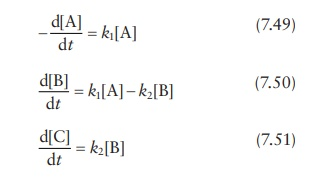The concentration time profiles for all species in this reaction can be obtained by simultaneously solving the above differential equations.

Contact Us, Privacy Policy, Terms and Compliant, DMCA Policy and Compliant

TH 2019 - 2022 pharmacy180.com; Developed by Therithal info.